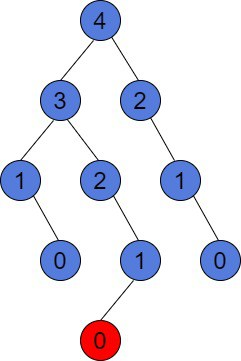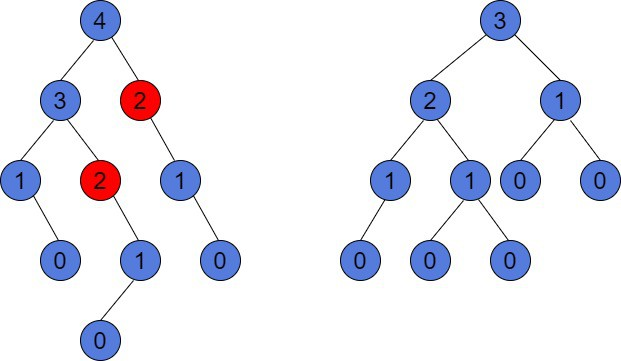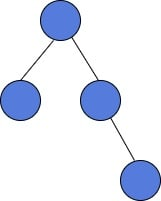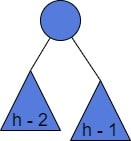## 1. Overview

In this tutorial, we’ll introduce a balanced Binary Search Tree. Moreover, we’ll learn about what is the height of a tree and show that in a balanced tree withnodes it is.

We’ll also use Big-O notation to approximate the tree height. We assume having a basic knowledge of Binary and Binary Search Trees.

## 2. Tree Height

The height of a tree is the longest downward path from its root to any reachable leaf.

Let’s look at an example:There is a height value in each node in the above tree. Notice that the longest path from the root node to the furthest leaf, colored in red, is 4. Hence the height of this tree is 4. To compute this, we can follow a simple algorithm.

We can traverse the tree with post-order traversal to compute the height of each node. At each algorithm step, we calculate current node’s height by adding 1 to, whereandare the height of the left and right subtree respectively. The height of a leaf is considered to be 0.

The time complexity of operations on Binary Search Trees (BST) are usually based on its height. Thus, a BST withnodes can be built such that the search operation will taketime. However, it’s considered to be inefficient, because BSTs can perform much better.

A BST is an ordered data structure. The search and delete operations costtime, whereis the height of the tree. The best case is when. However, the worst case is. Further, we’ll see that in a balanced BST,is always.

## 3. Balanced Binary Tree

A binary tree is balanced, if, for every node, the heights of its left and right children differ by at most 1. If a node doesn’t have any of the children, then the height of the absent children is -1. Let’s have a look at these two trees:In the tree on the left, nodes of a height 2, marked in red, make this binary tree unbalanced. The height of their left child, which is absent, is  -1. However, the height of their right child is 1. The difference is, which is greater than 1. However, we can reorder the nodes. The right tree is balanced, in case, for every node, the difference between its children’s height is at most 1.

The example of a balanced BST is a Red-Black-Tree. The Red-Black-Tree is self-balancing. Balanced BSTs are also implemented in several Java Collections. For instance, TreeMap and TreeSet in Java have a Red-Black-Tree as a backbone.

### 3.1. Height of a Balanced Binary Tree

Balanced BST allows keeping the operations efficient. In a balanced BST ofnodes, we may be sure, that the search operation will taketime. Actually, the search operation would taketime ifis the height of a Balanced BST. Moreover, we can prove that its height is.

## 4. Lower Bound of a Balanced Tree Size

To prove that, we can prove the inequality, which gives us the lower bound of the balanced binary tree size given a height. Letbe the minimum number of nodes in a tree of height.

For a balanced binary tree of height, the minimum number of nodes is greater, than:. Let’s prove this statement. To do it, we’ll use mathematical induction on.

### 4.1. Mathematical Induction Base Case

For the base induction casea balanced binary tree of height 1 has at least 2 nodes. It has a root and either its left or right child is present. Thus, we have, which is correct.

Similarly, for the casea balanced binary tree has at least 4 nodes. Let’s see an example:We have, which is also correct.

### 4.2. Inductive Step

Now, let’s prove the statement for the case. With the induction technique, we assume the statementholds for every value in the range 1, 2, …, h – 1. Our task is to prove it holds for.

Below, we use a tree offor the tree of height.

So, a balanced binary tree ofwith the minimum number of nodeshas a root and two subtrees. Since it has the minimum number of nodes, one subtree has a height equal to. It’s true because we compute the height of the root as. Therefore, one of the root’s subtrees must be of.

Let’s look at the picture below:Since that tree has the minimum number of nodes, the other subtree has a height equal to. It can’t be shorter, because the entire binary tree is balanced.

As a result, we have. It’s also clear the smaller the height, the fewer nodes the tree has and. Thus,. By the induction hypothesis. So,. Finally,and.

We proved that the minimum number of nodesin a balanced binary tree ofis always greater than. We’ll use this in the next section.

## 5. Balanced Tree Height with Big-O Notation

Now we’ll show the heightof a balanced binary tree withnodes is. Firstly, let’s show. Previously, we’ve proved. Taking logarithm on both sides, we getor. Thus,.

Is important to remember,is the minimum number of nodes in a balanced binary tree of. It means,, whereis the number of nodes of any balanced binary tree of. Similarly,. So,.

Finally, we’ve proved thatfor any balanced binary tree of. Therefore,is.

## 6. Conclusion

In this article, we’ve discussed balanced binary trees and proved that their height is. Moreover, we’ve learned the way of proving theorems with mathematical induction. Finally, we’ve noticed, that the time complexity of some operations on a binary tree is based on its height. Therefore, a balanced binary tree is more efficient.